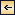Physical Properties of Sea Water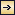### Thermal Properties of Sea Water

Thermal Expansion. The coefficient of thermal expansion, e, defined by e = (l/αs,ϑ,p)(∂αs,ϑ,p/∂ϑ), is obtained, at atmospheric pressure, from the terms for D in Knudsen's Hydrographical Tables, and at higher pressures from Ekman's tables or formulae (p. 57). The coefficient for sea water is greater than that for pure water and increases with increasing pressure. A few numerical values are given in table 9, in which negative values indicate contraction with increasing temperature.

COEFFICIENT OF THERMAL EXPANSION OF SEA WATER AT DIFFERENT TEMPERATURES, SALINITIES, AND PRESSURES (e × 106)
Pressure (decibars) Salinity ‰. Temperature (°C)
−2 0 5 10 15 20 25 30
0………… 0 −105 − 67 17 88 151 207 257 303
10 − 65 − 30 46 112 170 222 270 315
20 − 27 4 75 135 189 237 282 324
30 7 36 101 157 206 250 292 332
35 23 51 114 167 214 257 297 334
2,000………… 35 80 105 157 202 241 278
40 94 118 168 210 248 283
4,000………… 35 132 152 196 233 266
40 144 162 204 240 272
6,000………… 34.85 177 194 230
8,000………… 34.85 …… 231 246
10,000………… 34.85 …… 276 287

Thermal Conductivity. In water in which the temperature varies in space, heat is conducted from regions of higher to regions of lower

61
temperature. The amount of heat in gram calories per second which is conducted through a surface of area 1 cm2 is proportional to the change in temperature per centimeter along a line normal to that surface, and the coefficient of proportionality, κ, is called the coefficient of thermal conductivity (dQ/dt = −γ dϑ/dn). For pure water at 15°C the coefficient is equal to 1.39 ×10−3 The coefficient is somewhat smaller for sea water and increases with increasing temperature and pressure. This coefficient is valid, however, only if the water is at rest or in laminar motion (p. 89), but in the oceans the water is nearly always in a state of turbulent motion in which the processes of heat transfer are completely altered. In these circumstances the above coefficient of heat conductivity must be replaced by an “ceddy’ coefficient which is many times larger and which depends so much upon the state of motion that effects of temperature and pressure can be disregarded (p. 91).

Specific Heat. The specific heat is the number of calories required to increase the temperature of 1 g of a substance 1°C. When studying liquids, the specific heat at constant pressure, cp, is the property usually measured, but in certain problems the specific heat at constant volume, cv must be known.

The specific heat of sea water of different chlorinities was investigated by Thoulet and Chevallier, whose results have been recalculated and presented in different ways. Krümmel (1907) gives the following values for the specific heat at 17.5°C and atmospheric pressure: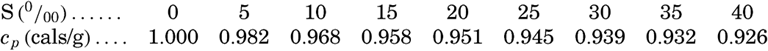[Equation]

Kuwahara (1939) gives an empirical equation for the specific heat at 0°C and atmospheric pressure: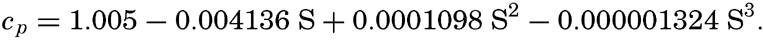[Equation]

It will be noted that the specific heat decreases with increasing salinity, but it has been pointed out by Krümmel that the effect is somewhat larger than might be expected from the composition of the solution, and the problem merits reinvestigation. The effects of temperature and pressure have not been measured, but it has been assumed that they are the same as those for pure water. Ekman (1914) gives the following values showing the effect of temperature on the specific heat of water of S = 34.85 ‰ at atmospheric pressure:[Equation]

The effect of pressure on the specific heat has been computed by Ekman (1914) from the equation

62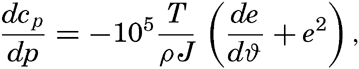[Equation]
where p is the pressure in decibars, T is the absolute temperature, ρ the density, J the mechanical equivalent of heat, and e the coefficient of thermal expansion. The combined effect of temperature and pressure is shown in table 10, where cp,0 is the specific heat at atmospheric pressure of water of salinity 34.85 ‰. The specific heat at constant volume, which is somewhat less than cp, may be computed from the following equation: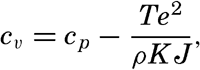[Equation]
where K is the true compressibility (p. 68) and where the other symbols are defined above. According to Matthews (1923), the ratio of cp,:cv, for water of S = 34.85 ‰ at atmospheric pressure increases from 1.0004 at 0° to 1.0207 at 30°. The effect of pressure is appreciable; for the same water at 0°, the ratio is 1.0009 at 1000 decibars, and 1.0126 at 10,000 decibars. This ratio is important in the study of the velocity of sound (P. 76).

DIFFERENCE BETWEEN SPECIFIC HEAT AT ATMOSPHERIC PRESSURE AND AT PRESSURE p, (cp,0cp,p) (Salinity 34.85 ‰ at indicated temperature)
θ (°C) p (decibars)
2000 4000 6000 8000 10,000
− 2………… 0.0171 0.0315 0.0435
0………… 0.0159 0.0291 0.0401 0.0492 0.0566
5………… 0.0136 0.0248 0.0340 0.0416 0.0479
10………… 0.0120 0.0220
15………… 0.0110 0.0203
20………… 0.0105

Latent Heat of Evaporation. The latent heat of evaporation of pure water is defined as the amount of heat in gram calories needed for evaporating 1 g of water, or as the amount of heat needed for producing 1 g of water vapor of the same temperature as the water. Only in the latter form is the definition applicable to sea water. The latent heat of evaporation of sea water has not been examined, but it is generally assumed that the difference between that and pure water is insignificant; therefore, between temperatures of 0° and 30°C, the formula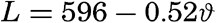[Equation]
can be used.

63

Adiabatic Temperature Changes. When a fluid is compressed, without gain or loss of heat to the surroundings, work is performed on the system and there is a rise in temperature. Conversely, if expansion takes place, the liquid itself gives up energy, which is reflected in a drop in temperature. Such adiabatic temperature changes are well known and important in the atmosphere. Sea water is compressible, and the effects of adiabatic processes, although small, must be taken into account when studying the vertical distribution of temperature in the great depths of the oceans and in deep isolated basins where the adiabatic heating may lead to a temperature increase toward the bottom (for example, p. 739). Adiabatic cooling is of immediate practical concern when water samples are taken with thermally insulated water bottles and the temperature of the water sample is determined after it has been raised to the surface (p. 355)

ADIABATIC TEMPERATURE GRADIENT IN THE SEA, IN °C PER 1000 M AT SALINITY 34.85‰
Depth (m) Temperature (°C)
−2 0 2 4 6 8 10 15 20
0. 0.016 0.035 0.053 0.078 0.087 0.103 0.118 0.155 0.190
1,000. 0.036 0.054 0.071 0.087 0.103 0.118 0.132 0.166 0.199
2,000. 0.056 0.073 0.089 0.104 0.118 0.132 0.146 0.177 0.207
3,000. 0.075 0.091 0.106 0.120 0.133 0.146 0.159 0.188
4,000. 0.093 0.108 0.122 0.135 0.147 0.159 0.170 0.197
5,000. 0.110 0.124 0.137 0.149
6,000. 0.120 0.140 0.152 0.163
7,000. …… 0.155 0.165 0.175
8,000. …… 0.169 0.178 0.187
9,000. …… 0.182 0.191 0.198
10,000. …… 0.194 0.202 0.209

Any adiabatic effect is related to changes in pressure, but in the sea the pressure can be considered proportional to the depth, and adiabatic temperature changes can be given as changes per unit of depth instead of per unit of pressure. According to Lord Kelvin, the change in temperature for each centimeter of vertical displacement is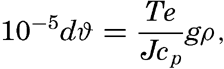[Equation]
where T is the absolute temperature and g is the acceleration of gravity, and where the other symbols have their previous meanings. This change
64
is extremely small, and for practical purposes the adiabatic temperature change on a vertical distance of 1000 m, called the adiabatic temperature gradient, is used instead. It should be observed that the adiabatic temperature gradient depends mainly upon the coefficient of thermal expansion, e, which varies much more with temperature and pressure than the other quantities involved. Ekman (1914) has computed the adiabatic temperature gradient for different temperatures, salinities, and depths, and some of his values are shown in table 11.

ADIABATIC COOLING (IN 0.01°C) WHEN SEA WATER (σ0 = 28.0, S = 34.85‰) WHICH HAS A TEMPERATURE OF ϑm AT THE DEPTH OP m METERS IS RAISED FROM THAT DEPTH TO THE SURFACE
Depth (m) Temperature, ϑm (°C)
− 2 −1 0 1 2 3 4 5 6 7 8 9 10
1,000…… 2.6 3.5 4.4 5.3 6.2 7.0 7.8 8.6 9.5 10.2 11.0 11.7 12.4
2,000…… 7.2 8.9 10.7 12.4 14.1 15.7 17.2 18.8 20.4 21.9 23.3 24.8 26.2
3,000…… 13.6 16.1 18.7 21.2 23.6 25.9 28.2 30.5 32.7 34.9 37.1 39.2 41.2
4,000…… 21.7 25.0 28.4 31.6 34.7 37.7 40.6 43.5 46.3 49.1 51.9 54.6 57.2
5,000…… 31.5 35.5 39.6 43.4 47.2 50.9 54.4
6,000…… 42.8 47.5 52.2 56.7 61.1 65.3 69.4
7,000…… …… …… 66.2 71.3 76.2 80.9 85.5
8,000…… …… …… 81.5 87.1 92.5 97.7 102.7
9,000…… …… …… 98.1 104.1 109.9 115.6 121.0
10,000…… 115.7 122.1 128.3 134.4 140.2

The temperature that a water sample would attain if raised adiabatically to the sea surface has been called the potential temperature (Helland-Hansen, 1912b) and has been designated Θ. Thus, Θ = ϑm − Δϑ, where ϑm, is the temperature in situ and Δϑ is the amount by which the temperature would decrease adiabatically if the sample were raised to the surface. The potential temperature can be obtained from a table of the adiabatic gradients by step-wise computations. Such computations are long and tedious, but Helland-Hansen (1930) has prepared a, convenient table for Δϑ which has been reproduced in table 12. The table is based on a salinity of 34.85 ‰ (σ0 = 28.0) and is applicable in general to the deep ocean areas, because in these the salinity does not differ much from 34.85 ‰ and because the effect of salinity on the adiabatic processes is small. It may be seen that if water of 2°C is raised adiabatically from 8000 m to the surface, Δϑ = 0.925°, and therefore the potential temperature of that water is 1.075°. The adiabatic cooling of water of different

65
salinities that may occur near the surface and in the Mediterranean Sea is given in tables 13 and 14.Physical Properties of Sea Water